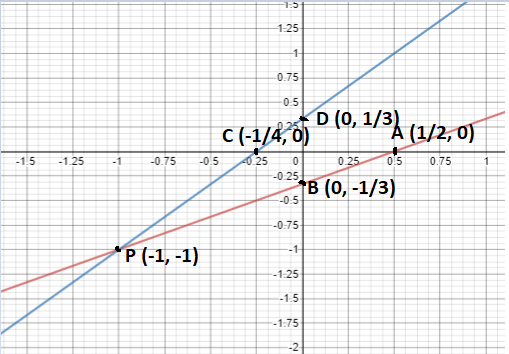Courses
Courses for Kids
Free study material
Free LIVE classes
MoreLIVE
Join Vedantu’s FREE Mastercalss

# Solve the following pair of equations graphically; 2x – 3y = 1 and 4x – 3y + 1 = 0A. x = 1, y = -1 B. x = -1, y = -1C. x = -1, y = 1D. x = 1, y = 1Verified
360.9k+ views
Hint: Let us plot the given equations in a coordinate plane to get the required value of x and y.

As we are asked to solve the given equations graphically.
So, we had to plot the equation on the graph.
For plotting a linear equation, we need at least 2 points through which the equation passes.
So, for our convenience we find those two points which lie on x-axis and y-axis and also satisfy the equation.
So, as we know that any point lying on the x-axis has y-coordinate equal to zero.
And, any point lying on the y-axis has x-coordinate equal to zero.
Given equations are,
2x – 3y = 1 (1)
4x – 3y + 1 = 0 (2)
So, let equation 1 passes through two points A (a, 0) and B (0, b).
And equation 2 passes through two points C (c, 0) and D (0, d).
Now finding the value of points A, B, C and D to plot them on the graph.
As point A (a, 0) lies on the equation 1. So, it must satisfy equation 1.
2a – 0 = 1
a = $\dfrac{1}{2}$
As point B (0, b) lies on the equation 1. So, it must satisfy equation 1.
0 – 3b = 1
b = $\dfrac{{ - 1}}{3}$
As point C (c, 0) lies on equation 2. So, it must satisfy equation 2.
4c – 0 + 1 = 0
c = $\dfrac{{ - 1}}{4}$
As point D (0, d) lies on equation 2. So, it must satisfy equation 2.
0 – 3d + 1 = 0
d = $\dfrac{1}{3}$
Now line joining points A$\left( {\dfrac{1}{2},{\text{ 0}}} \right)$ and B$\left( {0,{\text{ }}\dfrac{{ - 1}}{3}} \right)$ will be the equation 2x – 3y = 1.
And the line joining points C$\left( {\dfrac{{ - 1}}{4},{\text{ 0}}} \right)$ and D$\left( {0,{\text{ }}\dfrac{1}{3}} \right)$ will be the equation 4x – 3y + 1 = 0.Now we can see from the above graph that both the given lines intersect each other at P (-1, -1).
So, the solution of the given equation will be x = -1, and y = -1.
Hence, the correct answer will be B.

Note: Whenever we come up with this type of problem then to plot any line on a coordinate plane first, we should find points where the equation of line intersects x and y axis. And after plotting those points in the graph we will clearly see the intersection points of all the equations. And this will be the required solution of the problem.
Last updated date: 22nd Sep 2023
Total views: 360.9k
Views today: 3.60k